## Example Questions

### Example Question #31 : Triangles

Find the perimeter of an equilateral triangle with a height of.

Possible Answers: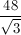None of the answer choices are correct.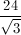Correct answer:Explanation:

Perimeter is found by adding up all sides of the triangle. All sides in an equilateral triangle are equal, so we need to find the value of just one side to know the values of all sides.

The height of an equilateral triangle divides it into two equal 30:60:90 triangles, which will have side ratios of 1:2:√3. The height here is the √3 ratio, which in this case is equivalent to 8, so to get the length of the other two sides, we put 8 over √3 (8/√3) and 2 * 8/√3 = 16/√3, which is the hypotenuse of our 30:60:90 triangle.

The perimeter is then 3 * 16/√3, or 48/√3.

### Example Question #2 : How To Find The Perimeter Of An Equilateral Triangle

If the height of an equilateral triangle is, what is the perimeter?

Possible Answers: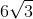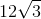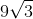Correct answer:Explanation:

By having a height in an equilateral triangle, the angle is bisected therefore creating two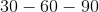triangles.

The height is opposite the angle. We can set-up a proportion.

Side oppositeis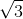and the side of equilateral triangle which is oppositeis.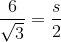Cross multiply.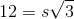Divide both sides by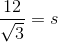Multiply top and bottom byto get rid of the radical.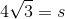Since each side is the same and there are three sides, we just multply the answer by three to get### Example Question #3 : How To Find The Perimeter Of An Equilateral Triangle

If area of equilateral triangle is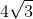, what is the perimeter?

Possible Answers:Correct answer:Explanation:

The area of an equilateral triangle is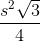.

So let's set-up an equation to solve for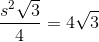Cross multiply.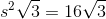Thecancels out and we get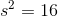.

Then take square root on both sides and we get. Since we have three equal sides, we just multplyby three to getas the final answer.

### All GRE Math Resources